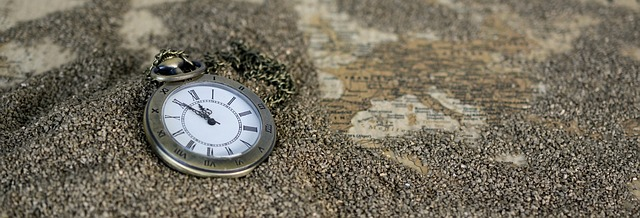## How do I set the time of java.util.Date instance to 00:00:00?The following code snippet shows you how to remove time information from the `java.util.Date` object. The static method `removeTime()` in the code snippet below will take a `Date` object as parameter and will return a new `Date` object where the hour, minute, second and millisecond information have been reset to zero. To do this we use the `java.util.Calendar`. To remove time information, we set the calendar fields of `Calendar.HOUR_OF_DAY`, `Calendar.MINUTE`, `Calendar.SECOND` and `Calendar.MILLISECOND` to zero.

``````package org.kodejava.util;

import java.util.Calendar;
import java.util.Date;

public class DateRemoveTime {
public static void main(String[] args) {
System.out.println("Now = " + removeTime(new Date()));
}

private static Date removeTime(Date date) {
Calendar calendar = Calendar.getInstance();
calendar.setTime(date);
calendar.set(Calendar.HOUR_OF_DAY, 0);
calendar.set(Calendar.MINUTE, 0);
calendar.set(Calendar.SECOND, 0);
calendar.set(Calendar.MILLISECOND, 0);
return calendar.getTime();
}
}
``````

The result of the code snippet above is:

``````Now = Sat Nov 20 00:00:00 CST 2021
``````

## How do I find the difference between two times?

The following code snippet show you how to find the difference between two time objects represented by `LocalTime` class. To get the difference between two `LocalTime` objects we can use the `Duration.between()` method. This method returns a `Duration` object, to get the difference in seconds we call the `getSeconds()` method.

Here a code snippet to demonstrate it.

``````package org.kodejava.datetime;

import java.time.*;

public class TimeDifference {
public static void main(String[] args) {
LocalTime start = LocalTime.now();
LocalTime end = LocalTime.of(16, 59, 55);
Duration duration = Duration.between(start, end);

System.out.printf("Seconds between %s and %s is: %s seconds.%n",
start, end, duration.getSeconds());

diffLocalDateTime();
diffInstant();
}

/**
* Difference between two LocalDateTime objects.
*/
public static void diffLocalDateTime() {
LocalDateTime dt1 = LocalDateTime.now();
LocalDateTime dt2 = LocalDateTime.now(ZoneId.of("GMT+0"));
Duration duration = Duration.between(dt1, dt2);
System.out.printf("Duration = %s seconds.%n", duration.getSeconds());
}

/**
* Difference between two Instant objects.
*/
public static void diffInstant() {
Instant instant1 = Instant.now();
Instant instant2 = Instant.EPOCH;
Duration duration = Duration.between(instant1, instant2);
System.out.printf("Duration = %s seconds.%n", duration.getSeconds());
}
}
``````

Using the `Duration.between()` we can also get the difference between two `LocalDateTime` objects and two `Instant` object as seen in the `diffLocalDateTime()` method and `diffInstant` method in the code snippet above.

The result of the code snippet:

``````Seconds between 15:05:35.401317900 and 16:59:55 is: 6859 seconds.
Duration = -28800 seconds.
Duration = -1637132736 seconds.
``````

## How do I format a message that contains time information?

Here we demonstrate how to use the `java.text.MessageFormat` class to format a message contains time information.

``````package org.kodejava.text;

import java.util.Date;
import java.util.Calendar;
import java.util.Locale;
import java.text.MessageFormat;

public class MessageFormatTime {
public static void main(String[] args) {
Date today = new Date();
Calendar calendar = Calendar.getInstance();

Date next7Hours = calendar.getTime();

// We want the message to be is Locale.US
Locale.setDefault(Locale.US);

// Format a time including date information.
String message = MessageFormat.format("Now is {0} and the next " +
"7 hours is {1}", today, next7Hours);
System.out.println(message);

// Format a time and display only the time portion
message = MessageFormat.format("Now is {0, time} and the next " +
"7 hours is {1, time}", today, next7Hours);
System.out.println(message);

// Format a time using a short format (e.g. HH:mm am/pm)
message = MessageFormat.format("Now is {0, time, short} and " +
"the next 7 hours is {1, time, short}", today, next7Hours);
System.out.println(message);

// Format a time using a medium format (eg. HH:mm:ss am/pm).
message = MessageFormat.format("Now is {0, time, medium} and " +
"the next 7 hours is {1, time, medium}", today, next7Hours);
System.out.println(message);

// Format a time using a long format (e.g. HH:mm:ss am/pm TIMEZONE).
message = MessageFormat.format("Now is {0, time, long} and the " +
"next 7 hours is {1, time, long}", today, next7Hours);
System.out.println(message);

// Format a time using a full format (e.g. HH:mm:ss am/pm TIMEZONE).
message = MessageFormat.format("Now is {0, time, full} and the " +
"next 7 hours is {1, time, full}", today, next7Hours);
System.out.println(message);

// Format a time using a custom pattern.
message = MessageFormat.format("Now is {0, time, HH:mm:ss.sss} " +
"and the next 7 hours is {1, time, HH:mm:ss.sss}", today, next7Hours);
System.out.println(message);
}
}
``````

The above program produces:

``````Now is 10/8/21, 9:38 PM and the next 7 hours is 10/9/21, 4:38 AM
Now is 9:38:10 PM and the next 7 hours is 4:38:10 AM
Now is 9:38 PM and the next 7 hours is 4:38 AM
Now is 9:38:10 PM and the next 7 hours is 4:38:10 AM
Now is 9:38:10 PM CST and the next 7 hours is 4:38:10 AM CST
Now is 9:38:10 PM China Standard Time and the next 7 hours is 4:38:10 AM China Standard Time
Now is  21:38:10.010 and the next 7 hours is  04:38:10.010
``````

## How do I convert string of time to time object?

You want to convert a string representing a time into a time object in Java. As we know that Java represents time information in a class `java.util.Date`, this class keep information for date and time.

Now if you have a string of time like `15:30:18`, you can use a `SimpleDateFormat` object to parse the string time and return a `java.util.Date` object. The pattern of the string should be passed to the `SimpleDateFormat` constructor. In the example below the string is formatted as `hh:mm:ss` (hour:minute:second).

``````package org.kodejava.util;

import java.text.DateFormat;
import java.text.SimpleDateFormat;
import java.util.Date;

public class StringToTimeExample {
public static void main(String[] args) {
// A string of time information
String time = "15:30:18";

// Create an instance of SimpleDateFormat with the specified
// format.
DateFormat sdf = new SimpleDateFormat("hh:mm:ss");
try {
// To get the date object from the string just called the
// parse method and pass the time string to it. This method
// throws ParseException if the time string is invalid.
// But remember as we don't pass the date information this
// date object will represent the 1st of january 1970.
Date date = sdf.parse(time);
System.out.println("Date and Time: " + date);
} catch (Exception e) {
e.printStackTrace();
}
}
}
``````

The code snippet above print the following output:

``````Date and Time: Thu Jan 01 15:30:18 CST 1970
``````